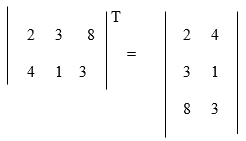## Pricing Table Particle

• Basic
• Standard Compliant Channels
• $50 • Completely synergize resource taxing relationships via premier market • 1 GB of space • Support at$25/hour
• Standard Compliant Channels
• $100 • Completely synergize resource taxing relationships via premier market • 10 GB of space • Support at$15/hour
• Platinum
• Standard Compliant Channels
• $250 • Completely synergize resource taxing relationships via premier market • 30 GB of space • Support at$5/hour

### Search Our Site

Matrix Algebra
Mathematics
Topic Fourteen

A Matrix is an array of numbers:

To add two matrices, just add the numbers in the matching positions: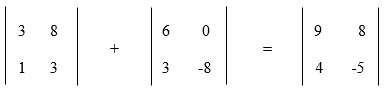These are the calculations: 3 + 6 = 9   8 + 0 = 8
1 + 3 = 4   3 – 8 = –5

The two matrices must be the same size, i.e. the rows must match in size, and the columns must match in size. For example, a matrix with 4 rows and 5 columns can be added to a matrix with 4 rows and 5 columns. However, the first matrix cannot be added to a matrix with 3 rows and 6 columns.

### Subtracting

To subtract two matrices: subtract the numbers in the matching positions: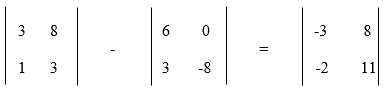These calculations are: 3 – 6 = –3   8 – 0 = 8
1 – 3 = –2   3 – (–8) = 11

### Multiply by a Constant

We can multiply a matrix by a constant (the value 3 in this case):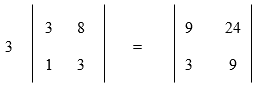These are the calculations: 3 x 3 = 9   3 x 8 = 24
3 x 1 = 3   3 x 3 = 9

The constant is called a scalar and the above procedure can be called scalar multiplication.

### Multiplying by another Matrix

To multiply two matrices together, the number of rows in the first matrix must equal the number of columns in the second matrix and the resulting answer will be a 2 by 2 matrix.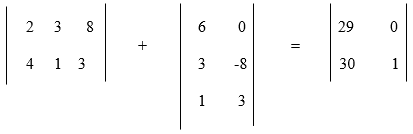The answer was obtained as follows:

(2, 3, 8) • (6, 3, 1) = 2x6 + 3x3 + 8x1 = 12 + 9 + 8 = 29

(2, 3, 8) • (0, -8, 3) = 2x0 + 3x-8+ 8x3 = 0 + -24 + 24 = 0

(4, 1, 3) • (6, 3, 1) = 4x6 + 1x3 + 3x1 = 24 + 3 + 3 = 30

(4, 1, 3) • (0, -8, 3) = 4x0 + 1x-8 + 3x3 = 0 + -8 + 9 = 1

### Dividing

In dividing matrices, we do not really divide, we do the following:

A/B = A × (1/B) = A × B-1

where B-1 means the inverse of B.

Therefore, instead of dividing, we multiply by an inverse.

### Inverse of a Matrix

The reciprocal is simply the 1 divided by the number.  For example, the reciprocal of 4 is 1/ 4.

The inverse of a matrix is the same idea but we write it B-1 or A-1.

When we multiply a number by its reciprocal we get 1

3 × (1/ 3) = 1

When we multiply a matrix by its inverse we get the Identity Matrix (which is like "1" for matrices):

A × A-1 = I

### Identity Matrix

We just mentioned the identity matrix. It is the matrix equivalent of the number "1":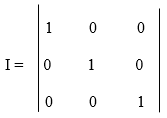The following are the properties of the above identity matrix:

• It is a 3x3 identity matrix
• It is "square" (has same number of rows as columns),
• It has 1s on the diagonal and 0s everywhere else.
• Its symbol is the capital letter I.

The inverse of A is A-1 only when:

A × A-1 = A-1 × A = I

The procedures for finding the inverse of a matrix:

1. Swap the positions of a and d and then place negatives in front of b and c, and divide everything by the determinant (ad-bc).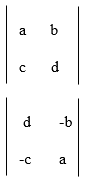2. Then find the determinant which is D = ad-bc.
3. Then find the inverse of the matrix and then multiply the converted matrix above by the inverse of the determinant.

Example

Find the inverse of the matrix:Solution

Swap the 7 and the 4 and put a negative sign by the 6 and 3 to get: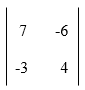Then find the determinant which is = 4 x 7 – 6 x 3 = 28 – 18 = 10.

Then multiply the converted matrix by 1/ 10 such as:which is the inverse matrix. When this inverse matrix is multiplied by the original matrix, the result should be the identity matrix such as follows: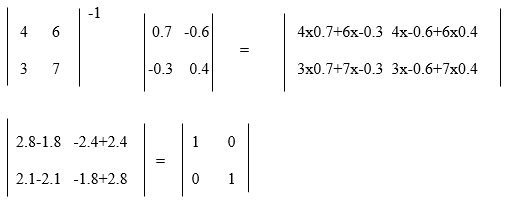So we ended up with the identity.

Transposing

In transposing a matrix, we swap or switch the rows and columns.

A “T” is placed in the top right-hand corner to mean transpose:

The transposition of the first matrix equals the second matrix.